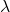## 580 nm light shines on a double slit with d=0.000125 m. What is the angle of the third dark interference minimum (m=3)? (Remember, nan

Question

580 nm light shines on a double slit with d=0.000125 m. What is the angle of the third dark interference minimum (m=3)?
(Remember, nano means 10^-9.)
(Unit=deg)

in progress 0
5 months 2021-09-04T07:29:23+00:00 1 Answers 4 views 0

## Answers ( )

0.66 degrees

Explanation:

The computation of the angle of the third dark interference is shown below:

The condition of the minima is

Path difference = (2n +1) ×÷ 2

For third minima, n = 2

Now

xd ÷ D = (2 × 2 + 1) ×÷ 2

d tan Q_3 = 5÷ 2

tan Q_3 = 5÷ 2d

Q_3 = tan^-1 × (5÷2d)

= tan^-1 × (5 × 580 × 10^-9) ÷ (2 × 0.000125)

= 0.66 degrees